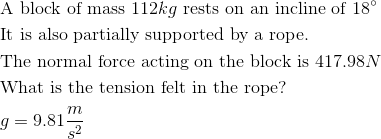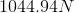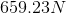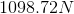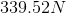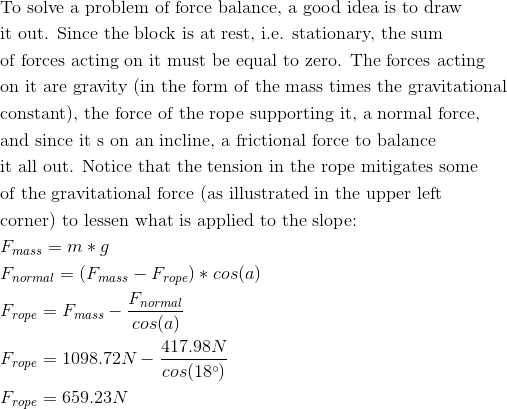# AP Physics C: Mechanics : Normal Force

## Example Questions

### Example Question #1 : Friction And Normal Force

Weightlessness is experienced when the normal force equals __________.

zero

a negative value

the force due to gravity

the mass of the object

zero

Explanation:

"Weightlessness" is analogous to free-fall (neglecting air resistance), during which the only force on an object is the force of gravity. When normal force becomes zero, the object loses physical contact with the surface.

When normal force is equal to the force of gravity, the object is in equilibrium and the net vertical force is zero. This results in zero acceleration, but does not result in "weightlessness." Mass is not a force, and negative force values merely indicate relative direction (assuming correct calculations)

### Example Question #2 : Friction And Normal Force

A book of mass 1kg is held to a vertical wall by a person's hand applying a 20N force directly toward the wall. The wall has a static friction coefficient of 0.3 and a kinetic friction coefficient of 0.2. With the book held at rest, what the is frictional force keeping the book from sliding down the wall?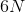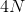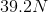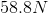Explanation:

Frictional force is calculated using the normal force and the friction coefficient. Since the book is at rest (not in motion), we use the coefficient of static friction.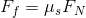The normal force will be equal and opposite the force pushing directly into the surface; in this case, that means the normal force will be 20N.

Solve for the force of friction: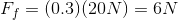### Example Question #3 : Friction And Normal Force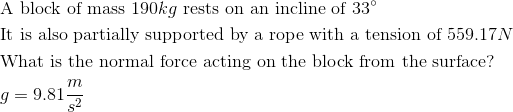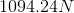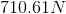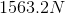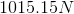Explanation: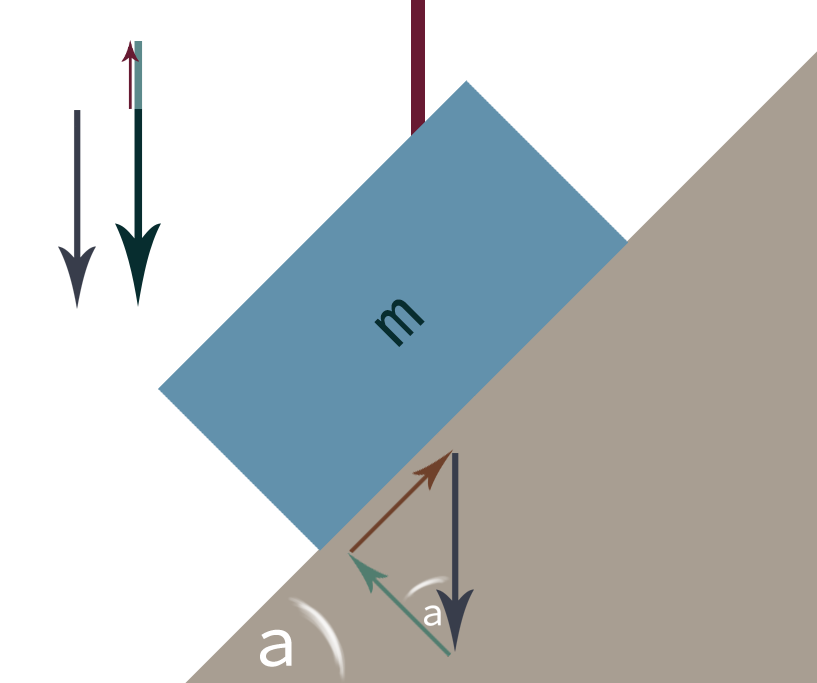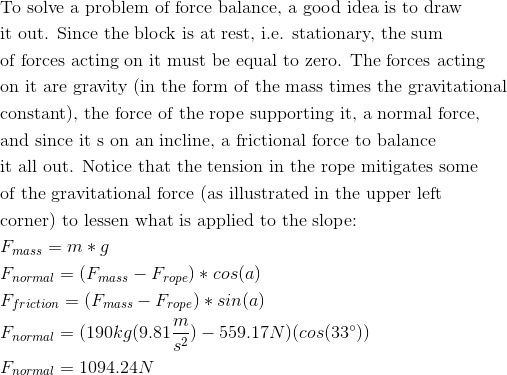### Example Question #4 : Friction And Normal Force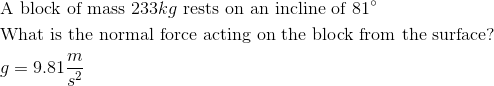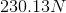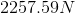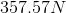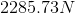Explanation: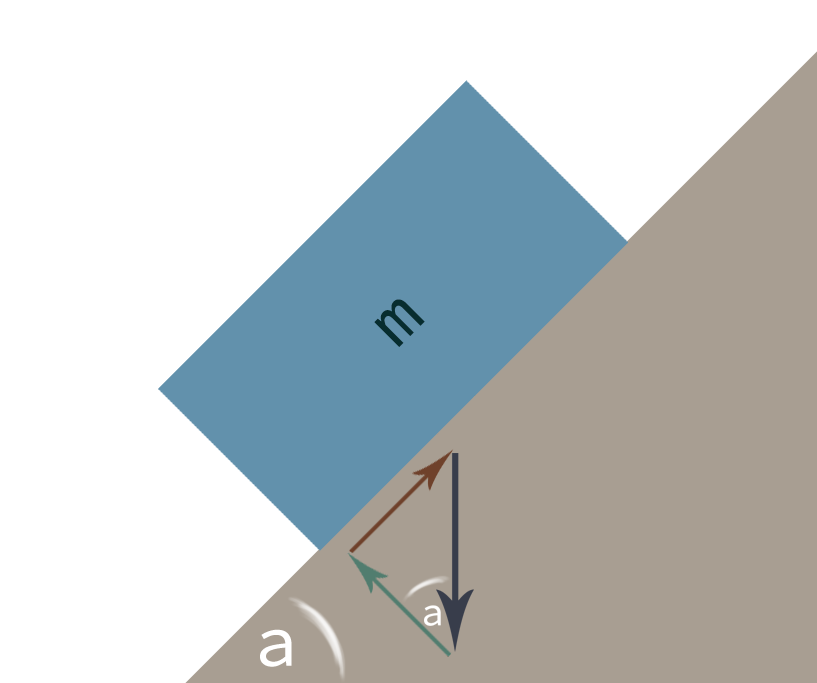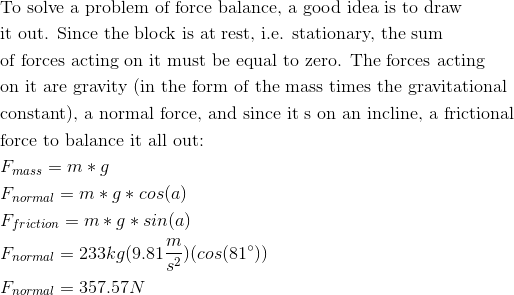### Example Question #5 : Friction And Normal Force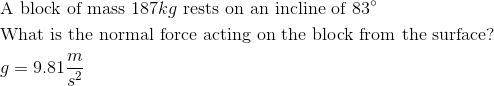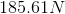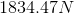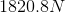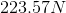Explanation: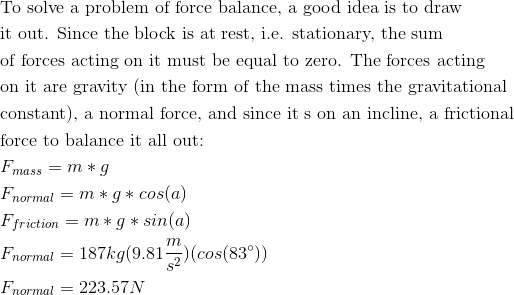### Example Question #6 : Friction And Normal Force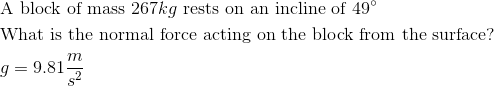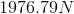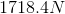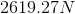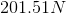Explanation: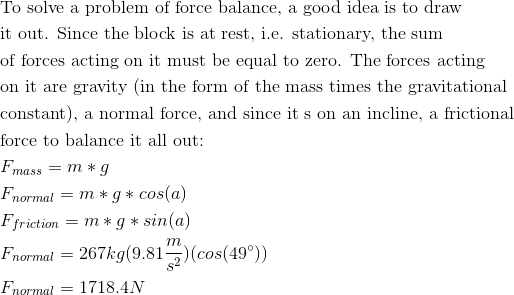### Example Question #7 : Friction And Normal Force